# AND Function in ExcelArticle byExcelMojo Team## What Is AND Function In Excel?

The AND function in Excel is a logical function that returns TRUE or FALSE values based on two or more conditions. It returns TRUE if all the logical values or conditions are true. The AND function is an inbuilt function.

For example, the below table shows the performance of employees. We can obtain the results using AND function in Excel. In the table,

• Column A displays the name of the employees.
• Column B shows the completed projects.
• The results should be displayed in column C.

The steps used to find results using the AND function in Excel are as follows:

• First, select an empty cell to display the output. In this example, let us select cell C2.
• We should enter the AND formula in cell C2.
• Let us check whether the values in cell B2 are greater than or equal to 50 (>=50).
• The AND Excel formula is =AND(B2>=50).
• Then, press Enter key to display the output.
• The AND function has returned the value as TRUE in cell C2.
###### Key Takeaways
• The AND function in Excel checks two or more conditions and returns TRUE if all the conditions are TRUE, else it returns FALSE.
• The formula is =AND(logical1,[logical2],…)
• logical1 is a mandatory argument and refers to the first logical value to be tested.
• logical2 is an optional argument; it denotes the second or the corresponding logical value.
• The function reads operators such as equal to, not equal to, greater than, less than, greater than or equal to, lesser than or equal to
• The function ignores blank values and returns #VALUE! error if the arguments have empty cells.

### AND( ) Excel Formula

The syntax of the AND function in Excel is:

The arguments of the AND function in the Excel are:

• logical1: It is a mandatory argument and refers to the first logical value to be tested.
• logical2: It is an optional argument; it denotes the second or the corresponding logical value.
##### Operators Used In AND Function

The AND formula in Excel involves some operators to perform certain tasks. The name and symbols of the operators are shown in the table below:

### How To Use AND Function In Excel?

There are two methods to use AND function in Excel. They are:

#### 1. Access From The Excel Ribbon

Follow the below steps to access AND function from the excel ribbon

1. Go to the Formulas tab.

2. Click on the Logical option from the Function Library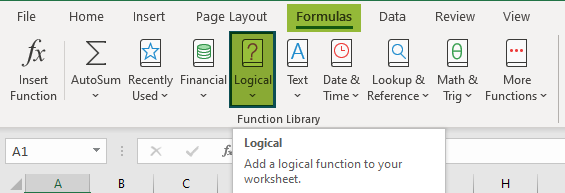3. Choose the AND function from the drop-down list.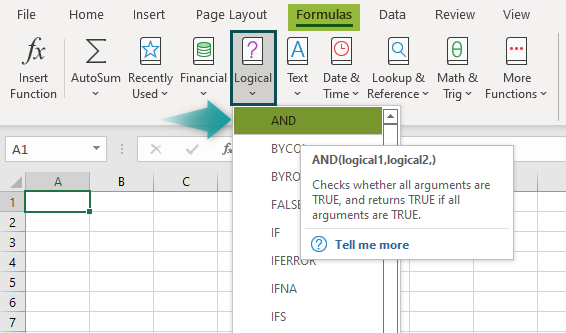4. The Function Arguments window pops up.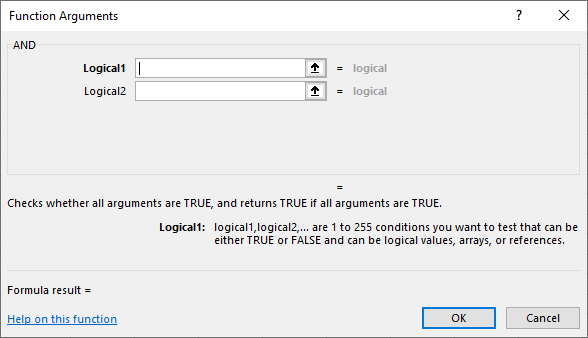5. Enter the arguments in the fields, Logical1 and Logical2 as seen above. Click OK.

#### 2. Enter the worksheet manually

• Select an empty cell to display the output. Type =AND( in the selected cell.

Alternatively, type =A, and double-click on the AND function from the list of suggestions shown by Excel.

• Enter the arguments as cell references or direct values.
• Close the parenthesis and press Enter key.

Let us understand the operators in AND function with an example.

##### Find Output Using Operators

The below table shows two values, V1 and V2, in columns A and B. We need to use the AND function in Excel to find the output using operators. The steps used to find results using the AND formula in Excel are as follows:

Step 1:  Select an empty cell to display the output. In this example, let us select cell C2.

Step 2:  We should enter the AND formula in cell C2.

Step 3: We should select the cell with the first value (first argument). In our example, we select cell A2.

Step 4: Let us check whether the values in cells A2 and B2 are equal or not using the equal to (=) operator.

Type = next to the first argument.

Step 5: Now, we should choose the corresponding second value (second argument). In our example, select cell B2.

The AND Excel formula is =AND(A2=B2).

Step 6: Press Enter key to display the output.

The AND function has returned the value as TRUE in cell C2.

• Similarly, by using the AND function, we can obtain the results.

### Examples

Let us understand how the AND function in Excel works with the following examples.

#### Example 1

Consider the below table listing the students’ scores in columns, A and B, respectively. We should find whether the students have scored greater than or equal to 60 or not using AND function in Excel.

The steps used to obtain results using the AND function are as follows:

Step 1:  We should select an empty cell to display the output. In this example, let us choose cell C2 in column C.

Step 2:  Next, we should enter the AND formula.

So, enter the formula in cell C2.

Step 3: We need to select the cell with the first value (first argument). In our example, let us choose cell B2.

Step 4: Let us check whether the entered values are greater than or equal to 60 (>=60).

Type >=60 next to the first argument.

The AND formula is: =AND(B2>=60).

Step 5: Press Enter key to display the output.

The AND function has returned the value as TRUE in cell C2.

Similarly, we can use the AND function in column C with the operator greater than or equal to 60 (>=60), or we can use the autofill option.

Step 6: Let us use the AutoFill option. Drag the cursor down to cell C6.

Excel automatically returns the values in cell range C3:C6 using the operator >=60, as shown in the image below.

The AND function has found that everyone except John has scored more than 60 with TRUE or FALSE conditions, where TRUE indicates the data which satisfies the condition, >=60, and FALSE shows those which do not satisfy the condition.

• Therefore, the AND function in Excel has evaluated the data and returned the output based on the condition (>=60).

#### Example 2 -Using IF function with AND function

The following table lists fruits along with the stock and demand details. We need to find the fruits with and without sales using IF and AND function in Excel.

In the table,

• Column A displays the fruits
• Column B shows the stock
• Column C indicates the demand for the fruits
• The results obtained should be displayed in column D

The steps used to obtain results using the IF and AND function in Excel are as follows:

Step 1:  We should select an empty cell to display the output. In this example, let us choose cell D2 in column D.

Step 2: Next, we should enter the formula.

We are using IF and AND function. So, we should enter =IF and AND formula in cell D2.

Step 3: We should select the cell with the first value (first argument). So, in our example, we need to select cell B2.

Step 4: Let us check whether the entered values are greater than or equal to 30 (>=30).

So, type >=30 next to the first argument.

Step 5: We need to select the corresponding second value (second argument). So, in our example, let us choose cell C2.

Step 6: We will also check whether the data is high in demand or not. High demand indicates that the fruit can be sold easily.

So, type = “High” in the formula and close the AND function with the bracket.

The IF function returns the value with TRUE or FALSE conditions. So, in this example, if both the conditions are TRUE, then the function returns “Sale” and returns the output as “No Sale” when the conditions are FALSE.

The complete formula is =IF(AND(B2>=30,C2=“High”),“Sale”,“No Sale”)

Step 7: Press Enter key.

The IF and AND function in Excel has returned the value as Sale in cell D2. We can use the same function in other cells or use the Autofill option.

Step 8: Let us use the AutoFill option. Drag the cursor down to cell D6. Excel automatically returns the values in cell range D3:D6 using the operator, >=60.

The AND with IF Excel functions have found that bananas have a high demand value and satisfies the condition, >=30. Also, the TRUE or FALSE conditions are indicated using ‘Sale’ and ‘No Sale’ conditions.

• Therefore, using IF and AND function, we can evaluate the data.

#### Example 3-Using OR function with AND function

The below table shows the number of available bedsheets (double and single) in the store. We need to follow the steps using OR and AND function in Excel to obtain the results.

In the table,

• Column A shows the total number of bedsheets
• Column B displays the available double bedsheets
• Column C displays the available single bedsheets
• The results obtained should be displayed in column D

The steps used to obtain results using OR and AND function in Excel are as follows:

Step 1:  We should select an empty cell to display the output. In this example, let us choose cell D2 in column D.

Step 2: Next, we should enter the formula.

We are using AND function with the OR function. So, we should enter AND formula in cell D2.

Step 3: We should select the cell with the first value (first argument). So, in our example, we need to select cell A2.

Step 4: Let us check whether the entered values are greater than or equal to 50 (>=50).

So, type >=50 next to the first argument.

Step 5: We need to select the corresponding second value (second argument). So, in our example, we need to select cell C2.

Step 6: We will also check whether the entered value in column B is greater than or equal to ‘20’ and the value in column C is greater than or equal to ‘15’ using OR function.

So, type OR(B2>=20),OR(C2>=15) in the formula and close the AND function with the bracket.

If both the conditions are true, OR with AND function returns TRUE; if the data does not satisfy the conditions, it returns FALSE.

The complete formula is =AND(A2>=50,OR(B2>=20),OR(C2>=15)).

Step 7: Press Enter key.

The OR with AND function has returned the value as FALSE in cell D2.

We can use the OR with AND function in other cells or we can use the autofill option.

Step 8: Let us use the AutoFill option. Drag the cursor down to cell D6.

Excel automatically returns the values in cell range D3:D6.

The OR with AND functions has returned the availability status using the conditions, >=20 and >=15.

• Likewise, the OR and AND function in Excel has evaluated the data.

### Important Things To Note

• The #VALUE! error occurs when an argument is blank or if no logical value is found.
• The maximum number of arguments was 30, and the maximum number of characters in a formula was 1,024 in Excel 2003 or older versions.
• The maximum capacity was increased in the 2007 version. From Excel 2007 versions, the AND function can test up to 255 arguments, and can the length of the formula was exceeded to 8,162 characters.
• The AND function tests the conditions and returns TRUE or FALSE values.

What is AND function in Excel?

The AND function in Excel tests data based on the conditions and checks whether both the conditions are true or false. It returns the result as TRUE or FALSE values.

The formula of AND in Excel is;

=AND(logical1,[logical2],…)

For example, consider the below table with the list of marks obtained by Bob in an examination. We need to use the following steps to calculate output using AND function in Excel.

In the table,

1. Column A displays the subjects
2. Column B shows the marks obtained by Bob.
3. The results obtained should be displayed in column C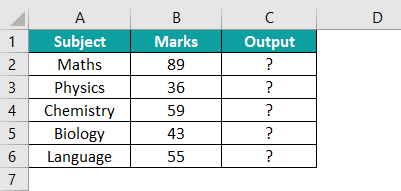The steps used to find the results using the AND function in Excel are as follows:

Step 1:  Select an empty cell to display the output. In this example, let us select cell C2.

Step 2:  We should enter the AND formula in cell C2.

Step 3: We should select the cell with the first value (first argument). So, in our example, we select cell B2.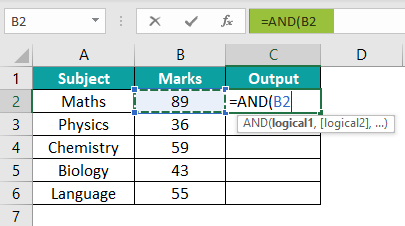Step 4: Let us check whether the values in cell B2 are greater than or equal to 45 (>=45).

So, the complete formula is: =AND(B2>=45).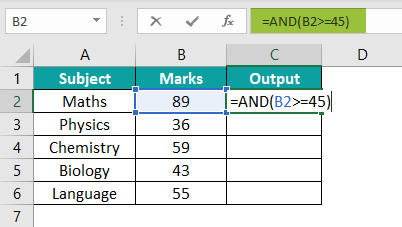Step 5: Press Enter key to display the output.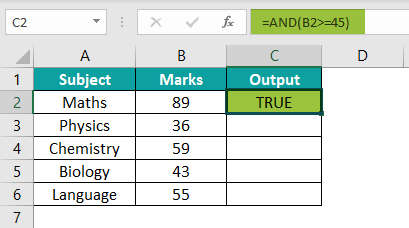The AND function has returned the value as TRUE in cell C2.

Similarly, we can use the AND function in other cells or we can use the autofill option to obtain the results.

Step 6: Let us use the AutoFill option. Drag the cursor down to cell C6.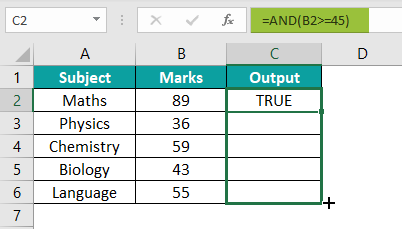Excel automatically returns the values in cell range C3:C6 using the operator >=45, as shown in the image below.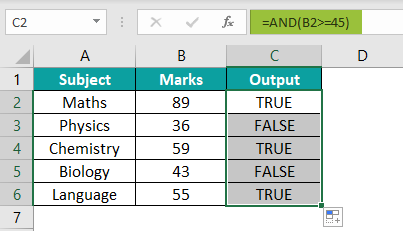Therefore, the AND function in excel has evaluated the data and returned the output based on the condition, (>=45).

Thus, we can find the AND function to find the output using operators.

When to use AND function in Excel?

The AND function in Excel is used when we have two or more conditions to test and wants the output value as TRUE or FALSE.

1. The function is used when several conditions need to be tested simultaneously.
2. It is used with the IF function when more than one condition must be tested.
3. The function is used with the OR function to compare two or more values.

Where is the AND function in Excel?

The AND function in Excel can be accessed using the following steps:
1. We should select the cell where we want to display the output.
2. Go to the Formulas tab.
3. Click on the Logical option from the Function Library group.4. Choose the AND function from the drop-down list.5. The Function Arguments window pops up.6. Enter the arguments in the Logical1 and Logical2 boxes.
7. Click OK.

Alternatively, we can also manually type the function in the desired cell.